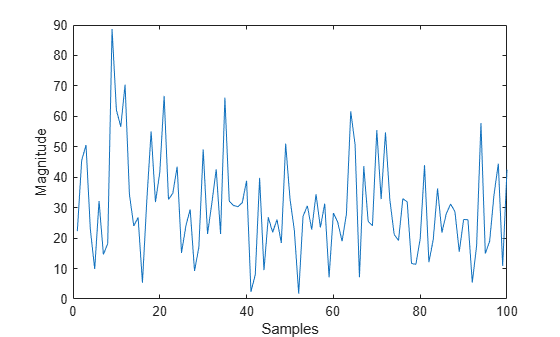# step

System object: barrageJammer

Generate noise jamming signal

## Syntax

```Y = step(H) Y = step(H,N) ```

## Description

Note

Starting in R2016b, instead of using the `step` method to perform the operation defined by the System object™, you can call the object with arguments, as if it were a function. For example, `y = step(obj,x)` and `y = obj(x)` perform equivalent operations.

`Y = step(H)` returns a column vector, `Y`, that is a complex white Gaussian noise jamming signal. The power of the jamming signal is specified by the `ERP` property. The length of the jamming signal is specified by the `SamplesPerFrame` property. This syntax is available when the `SamplesPerFrameSource` property is `'Property'`.

`Y = step(H,N)` returns the jamming signal with length `N`. This syntax is available when the `SamplesPerFrameSource` property is ```'Input port'```.

Note

The object performs an initialization the first time the object is executed. This initialization locks nontunable properties and input specifications, such as dimensions, complexity, and data type of the input data. If you change a nontunable property or an input specification, the System object issues an error. To change nontunable properties or inputs, you must first call the `release` method to unlock the object.

## Examples

expand all

Create a barrage jammer with an effective radiated power of 1000W. Then plot the magnitude of the jammer output. barrageJammer uses a random number generator. Plots can vary from run-to-run.

```jammer = barrageJammer('ERP',1000); plot(abs(jammer())) xlabel('Samples') ylabel('Magnitude')```## Version History

Introduced in R2021a